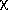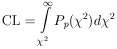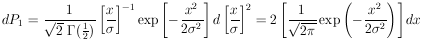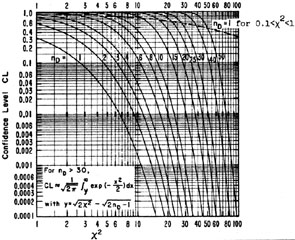### Appendix V. Cumulative Gaussian and Chi-Squared Distributions

The2 confidence limit is the probability of Chi-squared exceeding the observed value; i.e.,where Pp for p degrees of freedom is given by Eq. (30a).

Gaussian Confidence Limits

Let2 = [x /]2. Then for nD = 1,Thus CL for nD is twice the area under a single Gaussian tail. For example the nD = 1 curve for2 = 4 has a value of CL = 0.046. This means that the probability of getting | x|2is 4.6% for a Gaussian distribution.Figure 10.2 Confidence Level vs.2 for nD Degrees of Freedom (9).